Processing ......FreeComputerBooks.com Links to Free Computer, Mathematics, Technical Books all over the World

Semantics - Advances in Theories and Mathematical Models

• Title: Semantics - Advances in Theories and Mathematical Models
• Publisher: IN-TECH (April , 2012); eBook (Creative Commons Licensed)
• Hardcover: 284 pages
• eBook: PDF files and an Zipped PDF, 4.57 MB
• Language: English
• ISBN-10: N/A
• ISBN-13: 978-953-51-0535-0,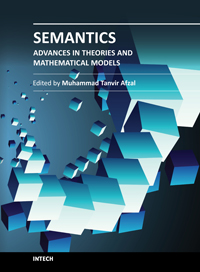Book Description

The current book is a nice blend of number of great ideas, theories, mathematical models, and practical systems in the domain of Semantics. The book has been divided into two volumes. The current one is the first volume which highlights the advances in theories and mathematical models in the domain of Semantics.

This volume has been divided into four sections and ten chapters. The sections include: 1) Background, 2) Queries, Predicates, and Semantic Cache, 3) Algorithms and Logic Programming, and 4) Semantic Web and Interfaces. Authors across the World have contributed to debate on state-of-the-art systems, theories, mathematical models in the domain of Semantics. Subsequently, new theories, mathematical models, and systems have been proposed, developed, and evaluated.

• N/A
Reviews, Ratings, and Recommendations: Related Book Categories: Read and Download Links:Similar Books:
•Semantics with Applications: A Formal Introduction

This book provides a needed introductory presentation of the fundamental ideas behind Semantics, stresses their relationship by formulating and proving the relevant theorems, and illustrates the applications of semantics in computer science.

•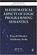Mathematical Aspects of Logic Programming Semantics

This book discusses applications of Logic Programming to computational logic and potential applications to the integration of models of computation, knowledge representation and reasoning, and the Semantic Web.

•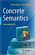Concrete Semantics: With Isabelle/HOL (Tobias Nipkow, et al.)

The book teaches the reader the art of precise logical reasoning and the practical use of a proof assistant as a surgical tool for formal proofs about computer science artefacts. It represents a formal approach to computer science, not just Semantics.

•Semantics in Action - Applications and Scenarios

This book is a nice blend of number of great ideas, theories, mathematical models, and practical systems in the domain of Semantics. It highlights highlights the state-of-the-art application areas in the domain of Semantics.

•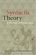Syntactic Theory: A Formal Introduction (Ivan A. Sag, et al)

This is a textbook that makes it truly fun to teach introductory syntax. It is thoroghly data-driven and teaches the student to pay attention to empirical details and to find linguistic patterns and explanations for them.

•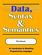Data, Syntax and Semantics (J. V. Tucker, et al.)

This book is an introduction to the mathematical theory of programming languages. It is in tended to provide a first course, one that is suitable for all university students of Computer Science to take early in their education.

•Foundations of Fuzzy Logic and Semantic Web Languages

This book provides a rigorous and succinct account of the mathematical methods and tools used for representing and reasoning with fuzzy information within Semantic Web languages. The book focuses on the three main streams of Semantic Web languages.

Book Categories
 :All CategoriesRecent BooksMiscellaneous BooksComputer LanguagesComputer ScienceData Science/DatabasesElectrical EngineeringJava and Java EE (J2EE)Linux and UnixMathematicsMicrosoft and .NETMobile ComputingNetworking and CommunicationsSoftware EngineeringSpecial TopicsWeb Programming
Other Categories